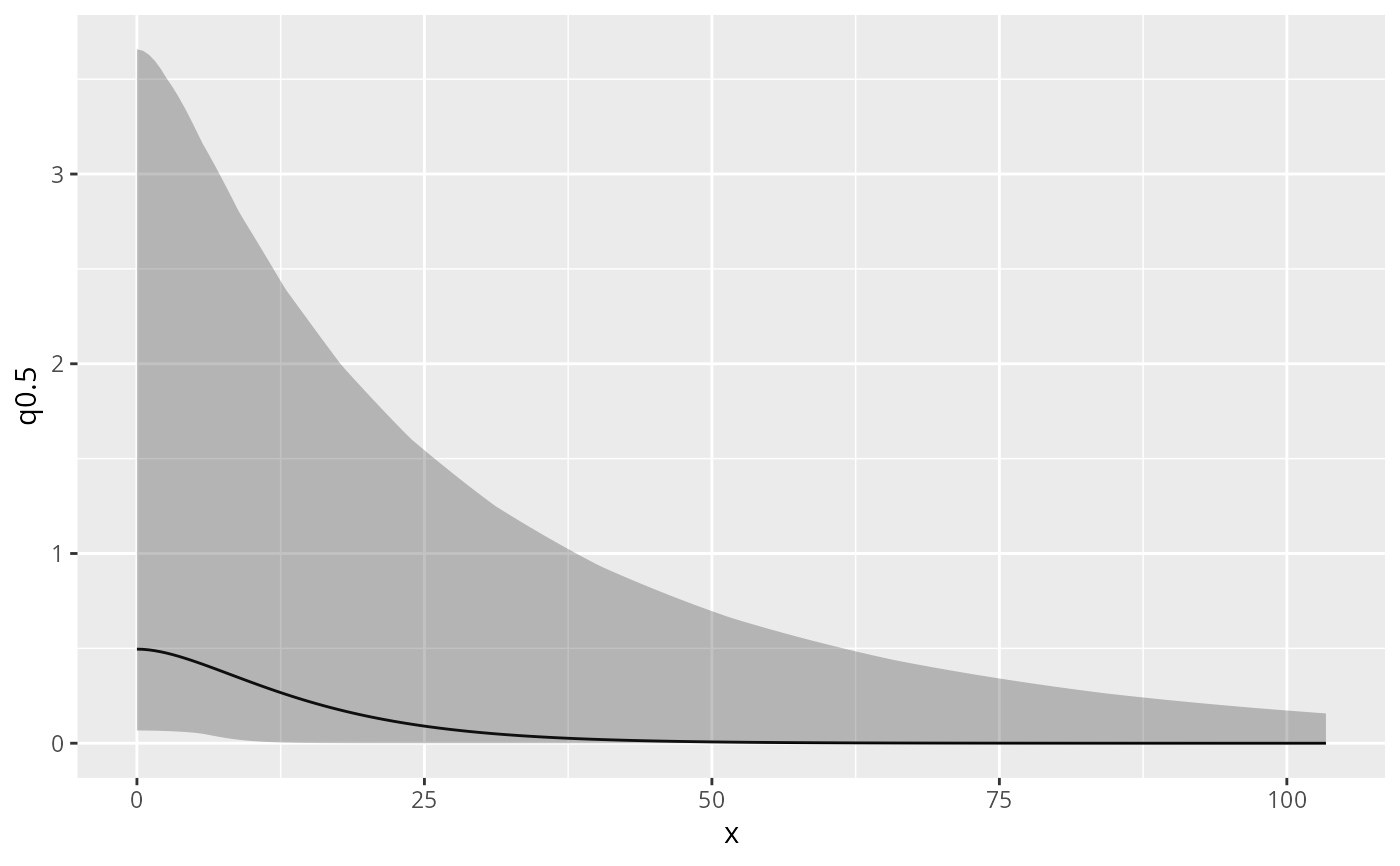Calculate posterior distribution of the range, log(range), variance, or log(variance) parameter of a model's SPDE component. Can also plot Matern correlation or covariance function. inla.spde.result.

## Usage

spde.posterior(result, name, what = "range")

## Arguments

result

An object inheriting from inla.

name

Character stating the name of the SPDE effect, see names(result\$summary.random).

what

One of "range", "log.range", "variance", "log.variance", "matern.correlation" or "matern.covariance".

## Value

A prediction object.

## Author

Finn Lindgren Finn.Lindgren@ed.ac.uk

## Examples

# \donttest{
if (bru_safe_inla() && require(ggplot2, quietly = TRUE)) {

# Load 1D Poisson process data

data(Poisson2_1D, package = "inlabru")

# Take a look at the point (and frequency) data

ggplot(pts2) +
geom_histogram(aes(x = x), binwidth = 55 / 20, boundary = 0, fill = NA, color = "black") +
geom_point(aes(x), y = 0, pch = "|", cex = 4) +
coord_fixed(ratio = 1)

# Fit an LGCP model with  and SPDE component

x <- seq(0, 55, length = 20)
mesh1D <- INLA::inla.mesh.1d(x, boundary = "free")
mdl <- x ~ spde1D(x, model = INLA::inla.spde2.matern(mesh1D)) + Intercept
fit <- lgcp(mdl, data = pts2, domain = list(x = mesh1D))

# Calculate and plot the posterior range

range <- spde.posterior(fit, "spde1D", "range")
plot(range)

# Calculate and plot the posterior log range

lrange <- spde.posterior(fit, "spde1D", "log.range")
plot(lrange)

# Calculate and plot the posterior variance

variance <- spde.posterior(fit, "spde1D", "variance")
plot(variance)

# Calculate and plot the posterior log variance

lvariance <- spde.posterior(fit, "spde1D", "log.variance")
plot(lvariance)

# Calculate and plot the posterior Matern correlation

matcor <- spde.posterior(fit, "spde1D", "matern.correlation")
plot(matcor)

# Calculate and plot the posterior Matern covariance

matcov <- spde.posterior(fit, "spde1D", "matern.covariance")
plot(matcov)
}
#> Warning: All covariate evaluations for 'Intercept' are NULL; an intercept component was likely intended.
#>   Implicit latent intercept component specification is deprecated since version 2.1.14.
#>   Use explicit notation '+ Intercept(1)' instead (or '+1' for '+ Intercept(1)').# }